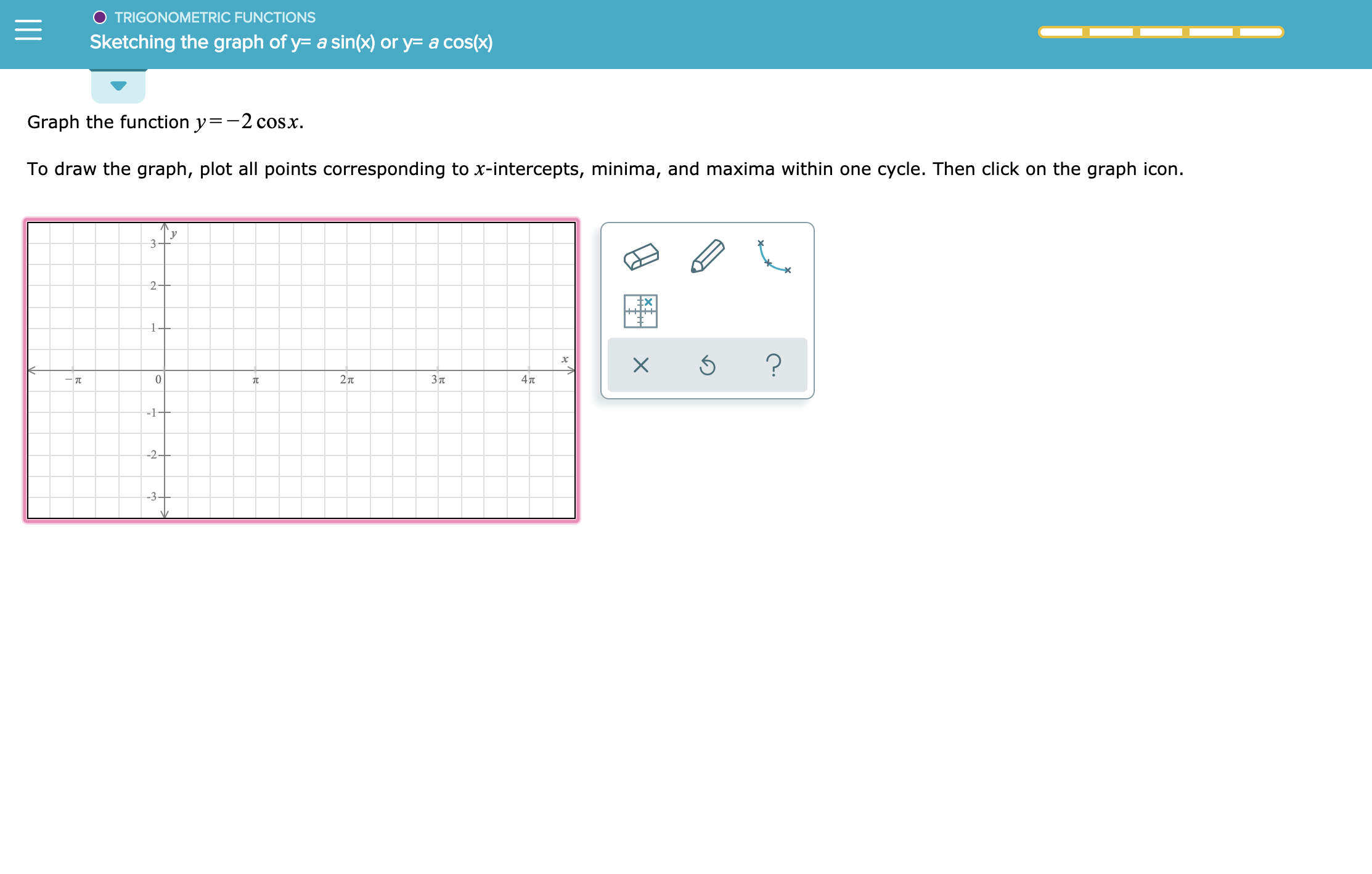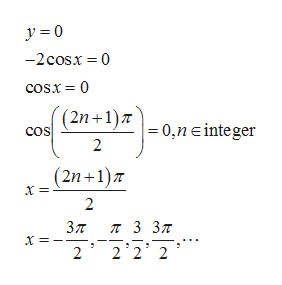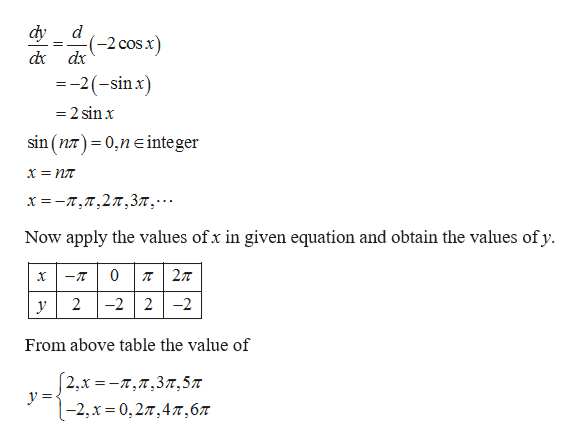# O TRIGONOMETRIC FUNCTIONSSketching the graph of y- a sin(x) or y= a cos(x)Graph the function y-2 cosxTo draw the graph, plot all points corresponding to x-intercepts, minima, and maxima within one cycle. Then click on the graph icon.2+?2737T4Tt-1--2+-3-

Question
1 views

see attachmenthelp_outlineImage TranscriptioncloseO TRIGONOMETRIC FUNCTIONS Sketching the graph of y- a sin(x) or y= a cos(x) Graph the function y-2 cosx To draw the graph, plot all points corresponding to x-intercepts, minima, and maxima within one cycle. Then click on the graph icon. 2+ ? 27 37T 4Tt -1- -2+ -3- fullscreen
check_circle

Step 1

x-intercepts:help_outlineImage Transcriptionclosey 0 -2cosx 0 cos.x 0 cos + 0,n einteger 2 (2n+1)T 2 Зл п 3 3л 2 2 2 2 fullscreen
Step 2

To find minima and maxima, differentiate the given equation with respect to x.help_outlineImage Transcriptionclosedy d (-2 cos.x) dx dx =-2(-sin x =2 sinx sin (n) 0,n einteger x= -π, π,2 π, 3π, . . . Now apply the values ofx in given equation and obtain the values ofy 0 -T 22 2 -2 From above table the value of 2,x -7,,37,57 y |-2,x 0,27,47,67 fullscreen
Step 3

Therefore, in one co...

### Want to see the full answer?

See Solution

#### Want to see this answer and more?

Solutions are written by subject experts who are available 24/7. Questions are typically answered within 1 hour.*

See Solution
*Response times may vary by subject and question.
Tagged in

### Other